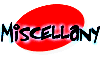Calculating speed from RPM

thanks to Unca Joel Walker

Calculating rpm and speed

Question:

I'm interested in calculating how fast my engine will rev in every gear, for a given speed, with different tires. Is there a formula relating tire size, engine speed and road speed?

the relationship between engine speed (rpm) and road speed (mph) is determined by the characteristics of the drivetrain, from the crankshaft to the tire contact patch. to calculate road speed for a given engine, you will need to know the gear ratio(s) and the final drive ratio, or the overall ratio, and the rolling circumference of the tires connected to the speedometer (usually the driven tires).

the rolling circumference will be the most difficult to determine; gear ratios can be found in your owner's manual or R&T data panel. to find the rolling circumference directly by measurement, put a chalk mark on the tire and the ground so that they line up. with the car loaded normally (say, half a tank of fuel and the driver aboard), roll the car for exactly 10 revolutions of the tire to average things out a bit. mark the ground again, lining up the chalk mark on the tire. use a steel tape measure to find the distance covered.

alternatively, you can look up the circumference in the table below, which lists some of the more popular tire sizes. this table was compiled from tire-industry standards for the rolling circumference of new tires at 50 km/h (31 mph); the rolling circumference will vary from brand to brand, with speed, load, inflation pressure and wear. even radials exhibit "growth" at high speeds. error limits for the numbers in this table are +1.5 percent and -2.5 percent.

as a last resort, you can estimate the rolling circumference from the tire-size designation. for example, a 195/60R-15 tire will have a section height of 60 percent of 195mm, or 117mm; in English units, 4.61 inches. add to this one-half of the 15-in. wheel diameter, and you get 12.11 in. multiply by 2pi gets the circumference using inch units; then dividing by 12 gets you to the circumference in feet.

the formula relating road speed and engine rpm is

mph = (rpm * cir) / (gear * final * 88)

where rpm = engine rpm
cir = tire cicumference, in feet
gear = gear ratio of your car
final = final drive ratio of your car
88 = combines several conversion factors

for example, the audi 90 quattro (R&T, december 1987). its tires are 195/60VR-14,so our calculated circumference works out to be 72.92 inches or 6.08 feet. its final drive is 4.11:1, its 5th gear is 0.79:1, so their product is 3.25 and the rest is a quick calculation away:

mph = (rpm * 6.08) / (3.25 * 88) = 0.0213 rpm

so, for instance, the audi will do 21.3 mph for every 1000 rpm in 5th gear.

to work the equation the other way, to find engine rpm for a given road speed, you want to solve for rpm:

rpm = (mph * gear * final * 88) / circumference

at 60 mph, for instance, this has our audi revving a calculated 2822 rpm, within a few percent of our road-test computer's 2750 rpm.

tire size circumference (feet)

185/60 R14 5.79
185/65 R14 5.97
185/70 R14 6.25
195/60 R14 5.91
195/65 R14 6.10
195/70 R14 6.36
205/55 R14 5.86
205/60 R14 6.02
205/70 R14 6.53
215/70 R14 6.66
------------end of article-------------

when i did mine, i did the 10x rolling tire chalk mark method, in a mall parking lot. my 195/??R14 tires came out to 6.30 feet circumference, and the bentley book provided the gear ratios:

reverse 3.67
1st 3.78
2nd 2.06
3rd 1.23
4th 0.83
final 4.86